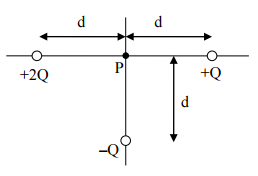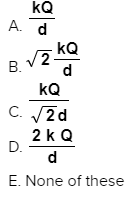# Problem: 1. What is the magnitude of the electric field at point P?2. What is the voltage at point P? (As always when dealing with point charges, we assume that the zero of voltage is at r = ∞.)

###### FREE Expert Solution

The electric field is expressed as:

$\overline{){\mathbf{E}}{\mathbf{=}}\frac{\mathbf{k}\mathbf{q}}{{\mathbf{r}}^{\mathbf{2}}}}$

Here, k is Coulomb's constant, q is the charge, and r is the distance between the charge and the point where the electric field is felt.

In this problem, r = d.

Electric potential:

$\overline{){\mathbf{P}}{\mathbf{=}}\frac{\mathbf{k}\mathbf{Q}}{\mathbf{d}}}$

1.

The electric field at point P is due to charge 2Q directed towards Q.

It is also due to charge Q directed towards +2Q.

And due to charge, -Q towards charge -Q

90% (73 ratings)###### Problem Details1. What is the magnitude of the electric field at point P?2. What is the voltage at point P? (As always when dealing with point charges, we assume that the zero of voltage is at r = ∞.)Frequently Asked Questions

What scientific concept do you need to know in order to solve this problem?

Our tutors have indicated that to solve this problem you will need to apply the Electric Field concept. You can view video lessons to learn Electric Field. Or if you need more Electric Field practice, you can also practice Electric Field practice problems.